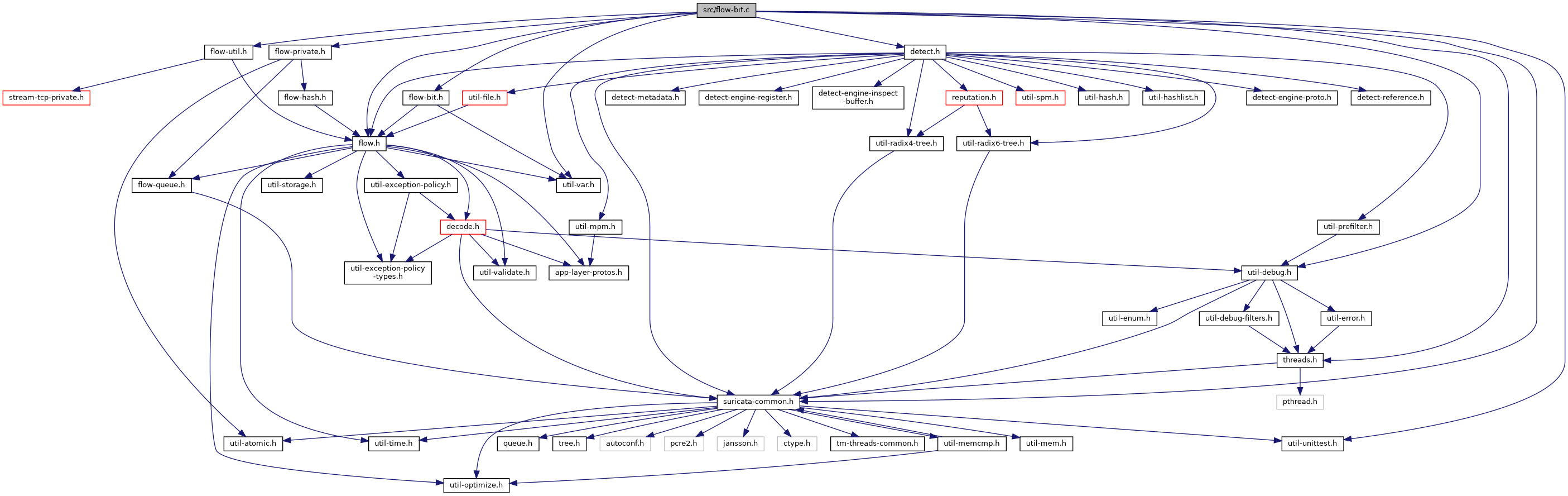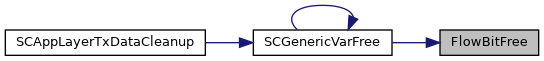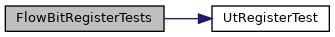suricata
flow-bit.c File Reference
`#include "suricata-common.h"`
`#include "threads.h"`
`#include "flow-bit.h"`
`#include "flow.h"`
`#include "flow-util.h"`
`#include "flow-private.h"`
`#include "detect.h"`
`#include "util-var.h"`
`#include "util-debug.h"`
`#include "util-unittest.h"`
Include dependency graph for flow-bit.c:Go to the source code of this file.

## Functions

void FlowBitSet (Flow *f, uint32_t idx)

void FlowBitUnset (Flow *f, uint32_t idx)

void FlowBitToggle (Flow *f, uint32_t idx)

int FlowBitIsset (Flow *f, uint32_t idx)

int FlowBitIsnotset (Flow *f, uint32_t idx)

void FlowBitFree (FlowBit *fb)

void FlowBitRegisterTests (void)

## Detailed Description

Implements per flow bits. Actually, not a bit, but called that way because of Snort's flowbits. It's a binary storage.

Todo:

move away from a linked list implementation

use different datatypes, such as string, int, etc.

have more than one instance of the same var, and be able to match on a specific one, or one all at a time. So if a certain capture matches multiple times, we can operate on all of them.

Definition in file flow-bit.c.

## Function Documentation

 void FlowBitFree ( FlowBit * fb )

Definition at line 128 of file flow-bit.c.

References Flow_::flowvar, GenericVarFree(), and SCFree.

Referenced by GenericVarFree().

Here is the call graph for this function:Here is the caller graph for this function:int FlowBitIsnotset ( Flow * f, uint32_t idx )

Definition at line 116 of file flow-bit.c.

Referenced by DetectFlowbitsRegister().

Here is the caller graph for this function:int FlowBitIsset ( Flow * f, uint32_t idx )

Definition at line 104 of file flow-bit.c.

Referenced by DetectFlowbitsRegister().

Here is the caller graph for this function:void FlowBitRegisterTests ( void )

Definition at line 402 of file flow-bit.c.

References UtRegisterTest().

Here is the call graph for this function:void FlowBitSet ( Flow * f, uint32_t idx )

Definition at line 84 of file flow-bit.c.

Referenced by DetectFlowbitsRegister().

Here is the caller graph for this function:void FlowBitToggle ( Flow * f, uint32_t idx )

Definition at line 94 of file flow-bit.c.

Referenced by DetectFlowbitsRegister().

Here is the caller graph for this function:void FlowBitUnset ( Flow * f, uint32_t idx )

Definition at line 89 of file flow-bit.c.

Referenced by DetectFlowbitsRegister().

Here is the caller graph for this function: